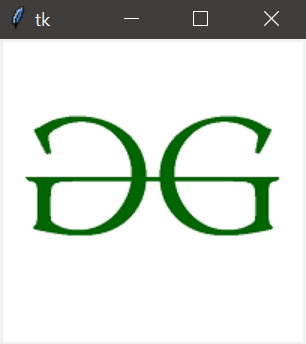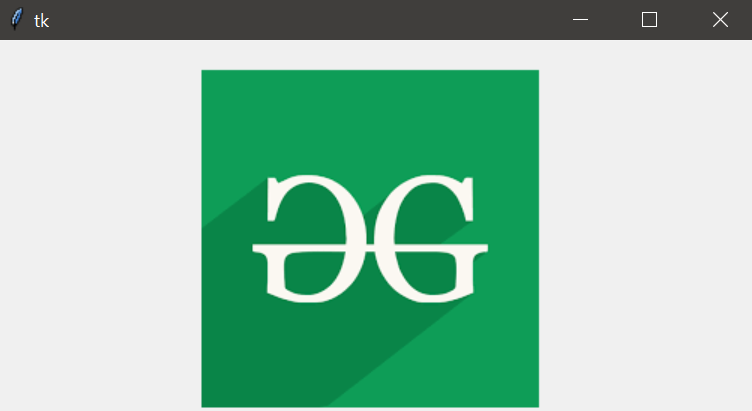Related Articles

# Reading Images With Python – Tkinter

• Difficulty Level : Easy
• Last Updated : 23 Mar, 2020

There are numerous tools for designing GUI (Graphical User Interface) in Python such as `tkinter`, `wxPython`, `JPython`, etc where Tkinter is the standard Python GUI library, it provides a simple and efficient way to create GUI applications in Python.

In order to do various operations and manipulations on images, we require Python `Pillow` package. If the `Pillow` package is not present in the system then it can be installed using the below command.

• In Command prompt:
`pip install Pillow`
• In Anaconda prompt:
`conda install -c anaconda pillow`

Example 1: The below program demonstrates how to read images with `tkinter` using `PIL`.

 `# importing required packages``import` `tkinter``from` `PIL ``import` `ImageTk, Image``import` `os`` ` `# creating main window``root ``=` `tkinter.Tk()`` ` `# loading the image``img ``=` `ImageTk.PhotoImage(Image.``open``(``"gfg.jpeg"``))`` ` `# reading the image``panel ``=` `tkinter.Label(root, image ``=` `img)`` ` `# setting the application``panel.pack(side ``=` `"bottom"``, fill ``=` `"both"``,``           ``expand ``=` `"yes"``)`` ` `# running the application``root.mainloop()`

Output:In the above program, an image is loaded using the `PhotoImage()` method and then it is read by using the `Label()` method.The `pack()` method arranges the main window and the `mainloop()` function is used to run the application in an infinite loop.

Example 2: Let us look at another example where we arrange the image parameters along with application parameters.

 `# importing required packages``import` `tkinter  ``from` `PIL ``import` `ImageTk, Image  `` ` `# creating main window``root ``=` `tkinter.Tk()  `` ` `# arranging application parameters``canvas ``=` `tkinter.Canvas(root, width ``=` `500``, ``                        ``height ``=` `250``)  `` ` `canvas.pack()  `` ` `# loading the image``img ``=` `ImageTk.PhotoImage(Image.``open``(``"gfg.png"``))  `` ` `# arranging image parameters ``# in the application``canvas.create_image(``135``, ``20``, anchor ``=` `NW,``                   ``image ``=` `img) `` ` `# running the application``root.mainloop() `

Output:In the above program, the application parameters are handled by using the `Canvas()` method and the image parameters are handled using `create_image()` method such that the image `gfg.png` is displayed in the main window having defined height and width .

Attention geek! Strengthen your foundations with the Python Programming Foundation Course and learn the basics.

To begin with, your interview preparations Enhance your Data Structures concepts with the Python DS Course. And to begin with your Machine Learning Journey, join the Machine Learning – Basic Level Course

My Personal Notes arrow_drop_up